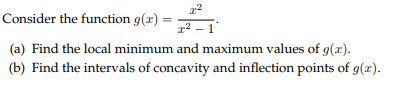# r2r2-1onsider the function q(rminimum and maximum values or q(b) Find the intervals of concavity and inflection points of g(x)

Question
6 viewshelp_outlineImage Transcriptioncloser2 r2-1 onsider the function q(r minimum and maximum values or q (b) Find the intervals of concavity and inflection points of g(x) fullscreen
check_circle

Step 1

(a). For any given function f(x), the local maximum or minimum exists at a point where x = a if f’(a) = 0

Applying derivative to the function with respect to x:

So for local maximum or minimum:

Hence, the local maximum or minimum exists at x = 0

Step 2

A point is said to be local maximum when f’'(x) < 0 and is said to be local minimum when f’'(x) > 0

Applying derivative to the first derivative:

Checking the value of this function at x = 0,

Since g''(0) < 0, local maximum exists at x = 0

Hence, the local maximum is given by:

Therefore, the local maximum value is 0 and the local minimum does not exist.

Step 3

(b)

A function f(x) has inflection points at x = a if f’'(a) = 0

Hence, the inflection point for the function g(x) is given by:

Hence,...

### Want to see the full answer?

See Solution

#### Want to see this answer and more?

Solutions are written by subject experts who are available 24/7. Questions are typically answered within 1 hour.*

See Solution
*Response times may vary by subject and question.
Tagged in

### Calculus